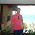As of May 4 2007 the scripts will autodetect your timezone settings. Nothing here has to be changed, but there are a few things

## Sunday, December 26, 2010

### Divisibility by 7

Let n be an integer with last digit d.
Repeat until divisibility of n by 7 has been decided:
- Set m to n with last digit removed.
- Set n to m - 2d
- Determine if n is divisible by 7.

Proof:
m = (n - d)/10
m = (n - d)/10 - 2d
m = ( n - 21d ) / 10
If 7 / n then 7 / ( n - 21d ) / 10 since (7,10)=1 and 7/21.

Example:
n = 8 641 969
m = 864 196
n = 864 196 - 18 = 864 178
m = 86 417
n = 86 417- 16 = 86 401
m = 8 640
n = 8640 - 2 = 8638
m = 863
n = 863 - 16 = 847
m = 84
n = 84 - 14 = 70
Divisible by 7.

I suppose trivia like this are only interesting for math(s)(*) enthusiasts and number geeks. Computational number theorists working with numbers of several million digits may actually use methods like this.

P.S.
(*) Just learned from Math is Fun that "Mathematics is commonly called Math in the US and Maths in the UK and in many other countries.".

#### 1 comment:

1.To know the first real rule for divisibility by 7 of the History of Number Theory watch this video:

## Welcome to The Bridge

Mathematics: is it the fabric of MEST?
This is my voyage
My continuous mission
To uncover hidden structures
To create new theorems and proofs
To boldly go where no man has gone before(Raumpatrouille – Die phantastischen Abenteuer des Raumschiffes Orion, colloquially aka Raumpatrouille Orion was the first German science fiction television series. Its seven episodes were broadcast by ARD beginning September 17, 1966. The series has since acquired cult status in Germany. Broadcast six years before Star Trek first aired in West Germany (in 1972), it became a huge success.)